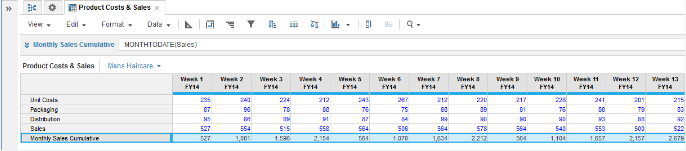1. Calculation functions
2. All Functions
3. Time and Date Functions
4. MONTHTODATEReturns a cumulative sums across a month time span for a single numeric parameter then resets.

## Syntax

`MONTHTODATE(x) `

where:

• x: line item to be cumulated

## Format

Input Format Output Format

x: Number

Matches the data format used by argument x

## Arguments

The function uses the following arguments:

• x: Number: Numeric line item, property, or expression

## Constraints

The function has the following constraints:

• Source Time Scale must be Day or Week.
• Cannot be used, if model timescale is General Weeks.

## Excel equivalent

• No Excel equivalent

## Example

MONTHTODATE is used to show cumulative sales figures for each month period for the first three month periods of a Weeks: 4-4-5 timescale in 2014:

The formula used in Monthly Sales Cumulative is:

`MONTHTODATE(Sales)`